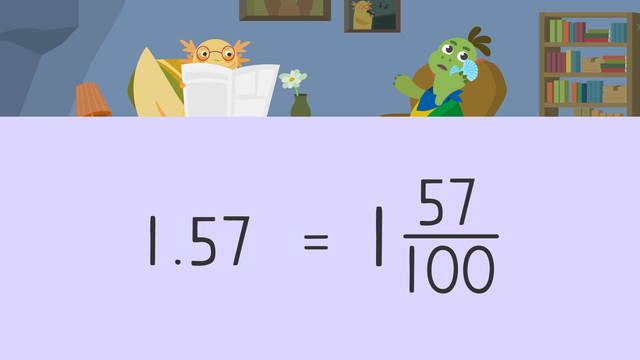# Decimals Greater than 1 as FractionsRating

Ø 5.0 / 2 ratings

The authorsTeam Digital
Decimals Greater than 1 as Fractions
CCSS.MATH.CONTENT.4.NF.C.6

## Basics on the topicDecimals Greater than 1 as Fractions

### Decimals Greater Than 1 as a Fraction Example

To write decimals greater than one as fractions, it is important to identify the place value for decimals greater than 1. This helps to know what the denominator is. Let’s look at the number one and fifty-seven hundredths.The first step is to set up a place value chart to the hundredths place, and write the number on the place value chart. This means one goes in the ones place, five goes in the tenths place, and seven goes in the hundredths place.Next, we write all the numbers as a fraction. One whole as a fraction is one over one. Five tenths as a fraction is five over ten, and seven hundredths as a fraction is seven over one hundred.Then, we need to convert the fractions to have common, or the same, denominators. In this example, we will convert all fractions to have the denominator one hundred. We multiply the numerator and denominator by one hundred for one over one to get one hundred over one hundred. Then, we multiply the numerator and denominator by ten for five over ten to get fifty over one hundred. Seven over one hundred does not need converting.Now, we add all the fractions together. The denominator remains one hundred, and we add one hundred plus fifty plus seven to give us one hundred and fifty-seven for the numerator.Since one hundred and fifty-seven is greater than one hundred, it is an improper fraction. We can rewrite this as the mixed fraction, one and fifty-seven over one hundred.This means that one and fifty-seven hundredths as a fraction is one and fifty-seven over one hundred.Below you will find a decimals greater than 1 worksheet to practice converting decimals greater than one to fractions.

### TranscriptDecimals Greater than 1 as Fractions

"Thanks for the help, Michelle." "Basically, we need to convert the decimals on your lock screen to fractions to unlock it!" "I just want to use my new shell phone." Let's help Axel unlock Tanks new shell phone by learning all about decimals greater than one as fractions. Decimals represent a whole number and a fractional part of a whole number. Decimals greater than one can be converted, or changed, into decimal fractions. Decimal fractions have a multiple of ten for the denominator, such as ten or one hundred. Let's look at the first decimal number to unlock Tank's new shell phone. We have one and fifty-seven hundredths. When converting decimal numbers to fractions, first, write each place value as a fraction. The ones place value is one over one, since it represents one whole. For the tenths place, write the decimal fraction five tenths, because it represents five equal parts of a whole divided into ten. For the hundredths place, write the decimal fraction seven hundredths, because it represents seven equal parts of a whole divided into one hundred. Next, convert to fractions with like denominators so the sum can be found. Convert all fractions to hundredths, the greatest denominator here. One over one becomes one hundred hundreths by multiplying the numerator and denominator by one hundred. Five tenths becomes fifty hundredths, by multiplying the numerator and denominator by ten. Seven hundredths already has one hundred for a denominator, so bring it down. Next, find the sum. The denominator stays one hundred. The sum of one hundred plus fifty plus seven, is one hundred fifty-seven. Convert to the mixed number one and fifty-seven over one hundred. One and fifty-seven hundredths is the same as one and fifty-seven over one hundred. Now the first code has been entered, let's look at the other code. The decimal number is three and four tenths. What is the first step? Write each place value as a fraction. Three as a fraction is three over one, and four tenths as a fraction is four tenths. What is the second step? Convert the fractions to fractions with like denominators. Since ten is the greatest denominator, convert the fractions to a denominator of ten. Three over one becomes thirty tenths, because we multiply the numerator and denominator by ten. And four tenths remains the same. What is the final step? Find the sum of the fractions. The denominator remains ten. Thirty plus four is thirty-four. Thirty-four tenths as a mixed number is three and four-tenths. Simplify further to three and two fifths by dividing the numerator and denominator by two. Three and four tenths is the same as three and two fifths! While Tank enters the last code to unlock his shell phone, let's review! Remember, to convert decimals greater than one to fractions, first, write each place value as a fraction. Second, convert the fractions to fractions with like denominators. Third, find the sum and simplify if needed. "And then my Mom was like, 'don't forget your turtleneck!', can you believe that?" "Oh boy, here we go...."